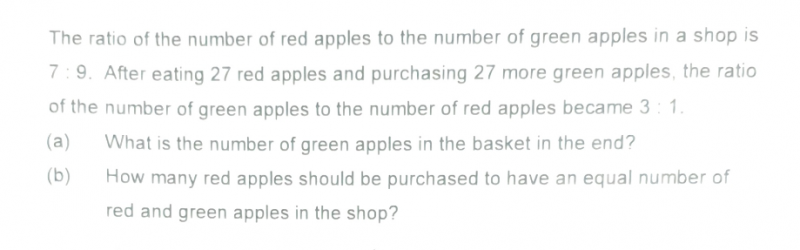# QuestionGd evening. Need help for my boy for this question. Tq.

Again this question is a bit mixed up…first shop then basket and then shop.  Also, they have already switched the ratio to trick the kids, that should be enough confusion in one question.

Red Apples                    Green Apples

Before            7u                                       9u

After                p                                         3p

(a)  p = 7u-27

3p = 9u+27 = 3(7u-27) =21u-81

21u-9u = 27+81 = 108  =>12u=108

u = 108/12 = 9     => p =7(9)-27 = 36

Green apples in the end (in the basket?) are 3p = 3 x36 = 108

(b) Since red apples = p = 36,

Red apples to purchase to equal green apples = 108-36 = 72

0 Replies 1 Like